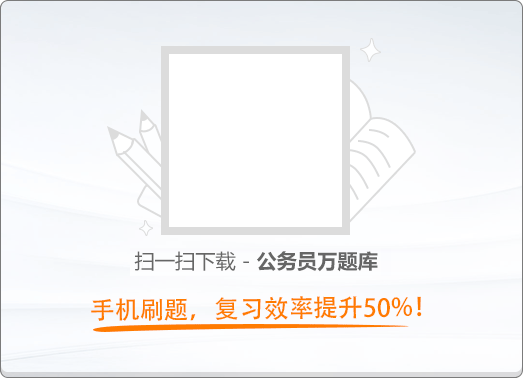120:002012年北京市公务员考试《行测》真题

1
(单项选择题)

• A.

科技强国

• B.

人才强国

• C.

文化强国

• D.

创新强国

• A
• B
• C
• D

2
(单项选择题)

• A.

持续稳定发展7%

• B.

持续稳定发展8%

• C.

平稳较快发展7.5%

• D.

平稳较快发展7%

• A
• B
• C
• D

3
(单项选择题)

• A.

家庭

• B.

指纹

• C.

婚姻

• D.

民族

• A
• B
• C
• D

4
(单项选择题)

• A.

符合落户条件的农业转移人口逐步转为城镇居民

• B.

促进农村转移人口城镇就业

• C.

合理控制大中城市的农业转移人口流入

• D.

鼓励和支持中西部地区、中小城市发展

• A
• B
• C
• D

5
(单项选择题)

• A.

江苏省

• B.

福建省

• C.

山东省

• D.

浙江省

• A
• B
• C
• D

6
(单项选择题)

2011年11月2日正式发布的“北京精神”的表述语为（　）。

• A.

爱党民主创新包容

• B.

爱国科学宽容厚德

• C.

爱国创新包容厚德

• D.

爱党宽容厚德创新

• A
• B
• C
• D

7
(单项选择题)

①追求真理是一个永无止境的过程

②人类对自然界的认识是一帆风顺的

③实践锻炼并提高了人的认识能力

④实践决定认识，认识是实践的目的

• A.

①②

• B.

①③

• C.

②④

• D.

③④

• A
• B
• C
• D

8
(单项选择题)

“苟利国家生死以，岂因祸福避趋之”。

• A.

热爱祖国，矢志不渝

• B.

天下兴亡，匹夫有责

• C.

维护统一，反对分裂

• D.

同仇敌忾，抵御外强

• A
• B
• C
• D

9
(单项选择题)

①发展对外经济关系必须坚持独立自主

②中国经济发展越来越吸引世界的目光

③发达资本主义国家主导世界经济格局的情况已经改变

④各国经济日益相互依赖、相互影响

• A.

①②

• B.

①③

• C.

③④

• D.

②④

• A
• B
• C
• D

10
(单项选择题)

2011年9月初，100欧元兑换人民币917.33元，2011年10月初，100欧元兑换人民币853.45元。

• A.

欧元购买力提高

• B.

我国出口到欧洲的产品竞争力上升

• C.

外币的汇率跌落

• D.

欧洲公民到我国旅游所需费用减少

• A
• B
• C
• D

11
(单项选择题)

• A.

勇于承担社会责任，完善失业保险制度

• B.

不断提高劳动生产率，提高企业竞争力

• C.

与员工协商薪酬，不断提高劳动者待遇

• D.

完善就业机制，加强员工职业技能培训

• A
• B
• C
• D

12
(单项选择题)

• A.

欧洲联盟

• B.

世界贸易组织

• C.

石油输出国组织

• D.

东南亚国家联盟

• A
• B
• C
• D

13
(单项选择题)

• A.

司法建议

• B.

查封

• C.

扣押

• D.

划拨

• A
• B
• C
• D

14
(单项选择题)

• A.

处罚

• B.

监督

• C.

强制执行

• D.

强制措施

• A
• B
• C
• D

15
(单项选择题)

• A.

过失杀人罪

• B.

故意杀人罪

• C.

遗弃罪

• D.

虐待罪

• A
• B
• C
• D

16
(单项选择题)

• A.

刑罚的轻重应当与犯罪行为相适应

• B.

刑罚的轻重应当与犯罪个人情况（主观恶性与人身危险性）相适应

• C.

刑罚的轻重应当与犯罪客观危害相适应

• D.

刑罚的轻重应当与犯罪分子所犯罪行和承担的刑事责任相适应

• A
• B
• C
• D

17
(单项选择题)

• A.

如实报道，不构成侵权

• B.

侵害了甲的名誉权

• C.

侵害了甲的姓名权

• D.

侵害了甲的身体权

• A
• B
• C
• D

18
(单项选择题)

• A.

甲用总面额1万元的假币参加赌博

• B.

甲是某银行工作人员，利用职务上的便利，用假币换取真币

• C.

甲在与乙签订经济合同时，为显示自己的经济实力，将总面额20万元的假币冒充真币出示给乙看

• D.

甲用总面额10万元的假币换取乙1万元的真币

• A
• B
• C
• D

19
(单项选择题)

• A.

发布在一定范围内应当遵守的事项

• B.

通告发布机关的级别一般比公告发布者低

• C.

告知的范围比公告宽泛

• D.

通告的文字具有强制力和严肃性

• A
• B
• C
• D

20
(单项选择题)

• A.

《××市人力资源和社会保障局关于加强城镇待业人员的管理的通知》

• B.

《××市人力资源和社会保障局关于对全市城镇待业人员进行统一登记的通知》

• C.

《××市人力资源和社会保障局关于进一步做好劳动就业工作的通知》

• D.

《××市人民政府关于加强劳动就业管理的公告》

• A
• B
• C
• D

21
(单项选择题)

• A.

民主共和思想

• B.

三权分立学说

• C.

君主立宪思想

• D.

天赋人权学说

• A
• B
• C
• D

22
(单项选择题)

• A.

丽泽

• B.

岳麓

• C.

石鼓

• D.

茅山

• A
• B
• C
• D

23
(单项选择题)

• A.

气温随高度的增加而递减

• B.

对流现象显著，多云雨现象

• C.

气流平稳，天气晴朗，有利于航空飞行

• D.

存在电离层，可反射无线电波

• A
• B
• C
• D

24
(单项选择题)

• A.

蒸汽机

• B.

轮船

• C.

汽车

• D.

电视机

• A
• B
• C
• D

25
(单项选择题)

• A.

A型血

• B.

B型血

• C.

AB型血

• D.

O型血

• A
• B
• C
• D

26
(多项选择题)

《北京市全民健身实施计划（2011—2015年）》提出，为实现目标任务，将要采取的措施有（　）。

• A.

强化政府公共服务职责，大力推进全民健身公共服务体系建设

• B.

加大科学健身指导力度

• C.

组织各项体育比赛活动，形成长效机制

• D.

促进全民健身服务业发展，引导城乡居民体育消费

• A
• B
• C
• D

27
(多项选择题)

• A.

老字号不仅是一种商贸景观，更重要的是一种历史传统文化现象

• B.

老字号是指具有鲜明的地域文化特征和历史痕迹、具有独特的工艺和经营特色的产品、技艺或服务

• C.

京城民间经常使用的歇后语，如东来顺的涮羊肉——真叫嫩、同仁堂的药——货真价实等，生动地反映了北京老字号的品牌特色

• D.

老字号随着历史的变迁和人们生活水平的不断提高，会逐渐消失

• A
• B
• C
• D

28
(多项选择题)

• A.

批准省、自治区和直辖市的建置

• B.

撤销下一级人民政府不适当的决定

• C.

决定特别行政区的设立及其制度

• D.

选举最高人民法院院长

• A
• B
• C
• D

29
(多项选择题)

• A.

• B.

• C.

• D.

民族乡

• A
• B
• C
• D

30
(多项选择题)

• A.

政府部门人员因公出国、出境费用支出

• B.

公务车购置及运行费用支出

• C.

政府部门购置办公设备费用支出

• D.

公务招待费用支出

• A
• B
• C
• D

31
(多项选择题)

• A.

主送机关即受文机关

• B.

印发机关指发文机关的办公部门

• C.

发文字号由发文机关代字、发文年度、发文序号组成

• D.

附件是附在正文之后的文件、材料，每个文件都有附件

• A
• B
• C
• D

32
(多项选择题)

• A.

国民收入总额及其增长速度

• B.

国民收入中积累和消费的比例

• C.

外贸状况特别是进口商品总量

• D.

人口总量

• A
• B
• C
• D

33
(多项选择题)

• A.

人民币升值给国内消费者带来的最明显变化，就是手中的人民币“更值钱”了，所以人民币升值让老百姓得到了更多实惠

• B.

人民币适当升值，能够迫使出口企业提高技术水平，改进产品档次，从而促进我国的产业结构调整，改善我国在国际分工中的地位

• C.

中国是个需求很大的大市场，所以，人民币升值对来中国投资的外商不会产生实质性的不利影响

• D.

人民币升值对出口企业和境外直接投资的影响，最终将体现在就业上

• A
• B
• C
• D

34
(多项选择题)

《民法通则》中，对公民民事行为能力进行分类的依据包括公民的（　）。

• A.

年龄状况

• B.

教育程度

• C.

家庭状况

• D.

精神状态

• A
• B
• C
• D

35
(多项选择题)

• A.

犯罪主体

• B.

犯罪主观方面

• C.

犯罪客体

• D.

犯罪客观方面

• A
• B
• C
• D